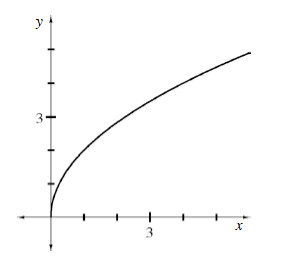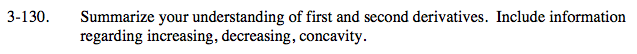### Home > CALC > Chapter 3 > Lesson 3.3.4 > Problem3-130

3-130.Summarize your understanding of first and second derivatives. Include information regarding increasing, decreasing, concavity. Homework Help ✎When a function f(x) is increasing and concave up: f '(x) > 0 and f "(x) > 0.
When a function f(x) is increasing and concave down: f '(x) _________ 0 and f "(x) __________ 0.
When a function f(x) is decreasing and concave up: f '(x)_________ 0 and f "(x) __________ 0.
When a function f(x) is decreasing and concave down: f '(x) _________ 0 and f "(x) __________ 0.